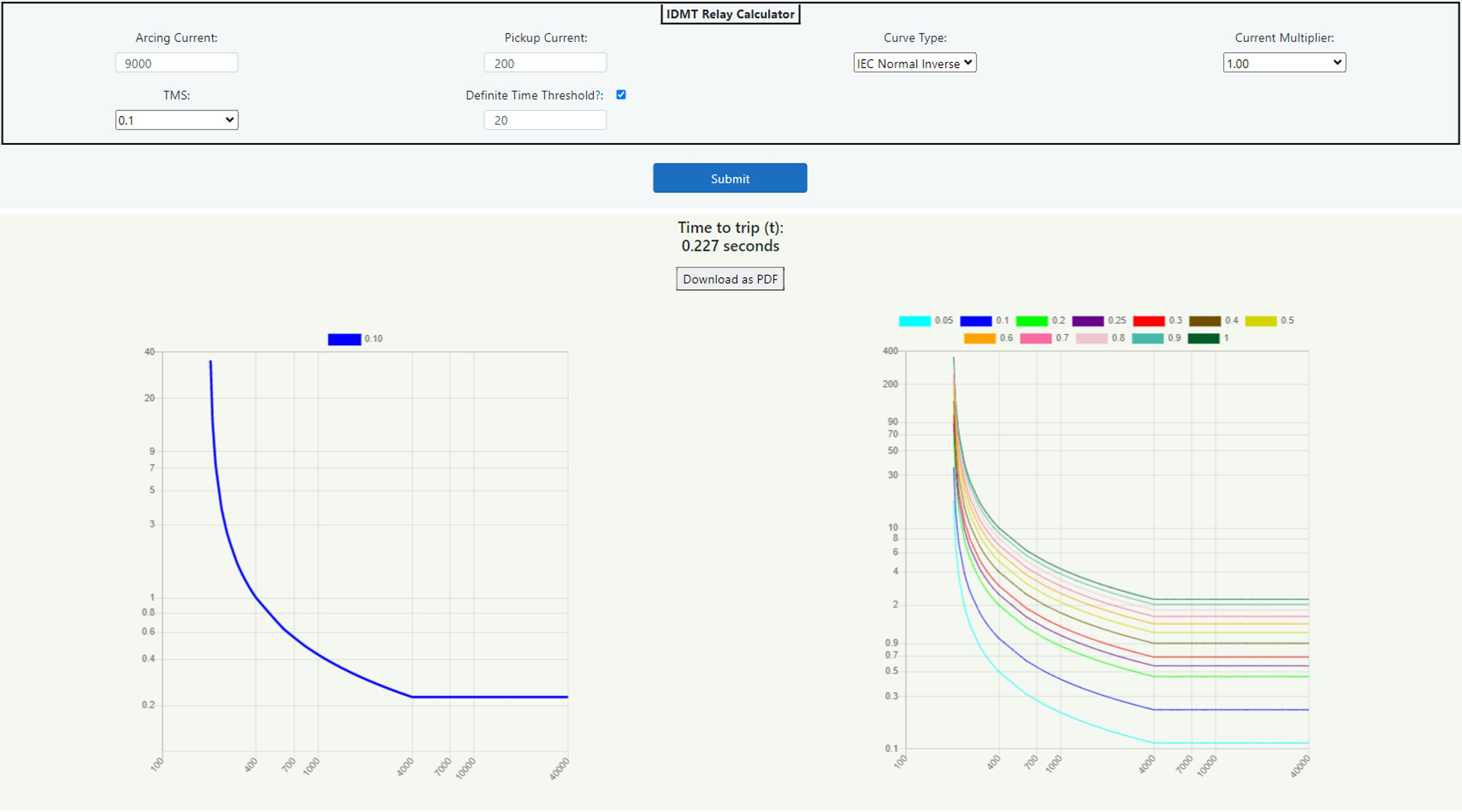14.11 IDMT Time Current Curve Calculator

The generic Inverse Definite Minimum Time (IDMT) time current curve calculator will allow you to not only produce curves for standard IEC and IEEE relay characteristics but will give a trip time for a given arcing current.

The standard IDMT overcurrent characteristics are expressed as a mathematical formula according to the IEC 60255-3/BS142 standards and IEEE C37.112-1996 and are often the basis of the electromechanical time current curves. The fact that the curves are based on a mathematical formula makes it easy to sense check the relay settings and of course electronic relays use the same curves.

The equation for operating time from IEC 60255 trip curves is as follows.

t(I) = TMS {
k
(
 I Is
) α - 1
}

Where, Is = the current setting and I = the actual current and k and α are the curve type constants. See table below.

There are four curve types used in IEC 60255 which are: standard inverse, very inverse, extremely inverse and long time standard inverse. For electromechanical relays, the curves are fixed for the particular relay model. So, in other words, if a standard inverse function is required then that is built into the specification of the relay. It will be necessary therefore to check the relay model with manufacturer’s data to determine the curve type.

 CURVE TYPE Constant k a Standard Inverse 0.14 0.02 Very Inverse 13.5 1 Extremely Inverse 80 2 Long Time Standard Inverse 120 1

Table 14.30 IEC Curve Constants

Note that US relay characteristics to ANSI and IEEE follow a similar format to the above equation but have a further constant B which is added to the expression.

The equation for operating time from IEEE C37.112-1996 trip curves is as follows.

t(I) = TD{
A
(
 I Is
)P - 1
+ B}

Where, Is = the current setting and I = the actual current and A, B and p are the curve type constants. TD stands for time dial and is often referred to as a time dial multiplier or TDM. The relationship is:

TDM =
 TD 7

See the below table.

 CURVE TYPE Constant A B p IEEE Moderately Inverse 0.0515 0.114 0.02 IEEE Very Inverse 19.61 0.491 2 IEEE Extremely Inverse 28.2 0.1217 2

Table 14.31 IEEE Curve ConstantsFigure 14.32 IDMT Time Current Calculator